# Differential and Common-Mode Voltages and Currents

On a two-wire transmission circuit, the difference between the instantaneous voltages a and b on the two wires is defined as the differential voltage d :

Equation 6.2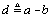where a and b are each measured with respect to a common arbitrary reference.

On a two-wire transmission circuit, the average of the instantaneous voltages a and b on the two wires is called the common-mode voltage c :

Equation 6.3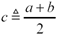where a and b are each measured with respect to a common reference, usually a local earth ground, but sometimes a local reference plane or other local reference point.

The differential and common-mode voltages comprise an alternate representation of the original signal, often called a decomposition of the original signal. Given the common-mode and differential voltages, you can reconstruct a and b . (The same decomposition applies to currents.)

Equation 6.4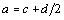Equation 6.5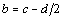In a good differential system one usually strives to limit the AC component of the common-mode signal. This is done because the common-mode portion of the transmitted signal does not enjoy any of the noise-canceling or radiation-preventing benefits of differential transmission. The common-mode and differential signals also propagate differently in most cabling systems, which can lead to peculiar skew or ringing problems if the common-mode component is an appreciable fraction of the overall signal amplitude, especially if those common-mode currents are accidentally converted into differential signals (see Section 6.8, "Differential to Common-Mode Conversion"). Intercabinet cabling, particularly, is extremely sensitive to the presence of high-frequency common-mode currents, which radiate quite efficiently from unshielded cabling.

Another decomposition of the two-wire transmission problem defines odd-mode and even-mode voltages and currents. These are similar to, but slightly different from, differential and common-mode voltages and currents.

An odd-mode signal is one that has amplitude x ( t ) on one wire and the opposite signal “ x ( t ) on the other wire. A signal with an odd-mode amplitude of x ( t ) has a differential amplitude of 2 x ( t ). If the signal x ( t ) takes on a peak-to-peak range of y , then the peak-to-peak odd-mode range is simply y , but the peak-to-peak differential amplitude is 2 y .

An even-mode signal is the same on both wires. An even-mode signal with a peak-to-peak range of y also has a peak-to-peak common-mode range of y . The even-mode amplitude and common-mode amplitude are one and the same thing.

Two-wire transmission systems sometimes send a signal voltage on one wire, but nothing on the other. In this case the differential-mode amplitude equals the signal amplitude on the first wire. The common-mode amplitude is half that value. In this case the odd-mode and even-mode amplitudes are the same and both equal to half the signal amplitude on the first wire.

Here are the translations between odd-mode and even-mode quantities . The same decomposition applies to currents.

Equation 6.6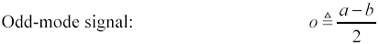Equation 6.7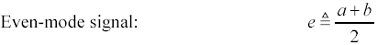Equation 6.8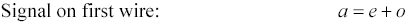Equation 6.9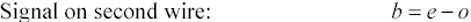where a and b represent the voltages on the two wires with respect to a common reference.

The differential-and-common-mode decomposition and the even-and-odd mode decomposition share very similar definitions. The discrepancy between the two models has to do with the definition of the differential mode. The differential voltage is what you read with an electrical instrument when you put it across two wires. The odd-mode voltage is a mathematical construct that simplifies the bookkeeping in certain situations.

Equation 6.10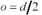Equation 6.11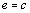Any noise like external RF interference that equally affects both wires of a differential pair will induce a common-mode (even-mode) signal, but not a differential-mode (odd-mode) signal (Figure 6.8). A good differential receiver senses only the differential signal and is therefore immune to this type of noise.

Figure 6.8. A good differential receiver cancels any noise that affects both wires equally, such as external RFI.POINTS TO REMEMBER

• Differential and common-mode signals are used to describe the voltages and currents on a two-wire transmission system.
• Odd-mode and even-mode signals are yet another way to describe the voltages and currents on a two-wire transmission system.
• Differential receivers cancel common-mode noise.High-Speed Signal Propagation[c] Advanced Black Magic
ISBN: 013084408X
EAN: N/A
Year: 2005
Pages: 163